## Visual Numbers: The Next Generation (I)

Hey, just because I’m an English major now doesn’t mean I can’t enjoy a bit of math on the side. Therefore, I present to you Visual Numbers: The Next Generation (Part I, insert obligatory Star Wars joke).

I’ve always been a big fan of fractals. I was imagining fractal-esque recursive structures back when solving 2x + 5 = 0 seemed intimidating. Recently, in my wanderings through the hallowed halls of physics, I stumbled across the idea of generating fractals by coloring each pixel of an image according to the final state of a differential equation, with the x and y coordinates of that pixel as input parameters. Of course, being an incompetent programmer, I haven’t been able to write a stable integrator for a differential equation, but I’m just smart enough to manage simple stuff like the discrete logistic map, where every new x is computed according to the formula x = λx(1-x), where λ is a constant. The other day, I had a brainstorm and decided to create a two-dimensional version of the same sort of thing, only with x as a vector and replacing x at each step with F(x), where F is a vector function. Basically, all that means is that I now have two integer variables to play with, which allows for more interesting behavior and allows me to create pretty pictures like this:In this image, each pixel is colored according to the following rule: all pixels start out white. Then, each pixel is turned into a vector v(x,y), where x and y are the pixel’s coordinates. The function v(x,y) = v(-x.y,x.x + floor(0.5 * x.y)) is then applied repeatedly for a fixed number of steps (in this case, 750). If at any point during iteration the vector reaches a previously-visited point, then that point forms a closed, repetitive orbit in 2-space and the pixel is colored black. If this doesn’t happen, the pixel is left white.

The shapes here are pretty nice, even if I do say so myself. From some prior experiments with similar mappings, I think that the smaller black ellipses with banding and satellite blobs represent orbits shaped like three elliptical orbits connected into a weird triangle, and that the large oval with the large banding represents the “spirograph-like” orbits.

The really neat thing about mappings like this is that they’re a (relatively) computationally-inexpensive alternative to the differential-equation mappings I discussed earlier. I’m no great mathematician (obviously), but I get the feeling that these two-dimensional mappings are discretized analogues of the mapping that generates the ever-beautiful Mandelbrot Set.

Interestingly (and here I’m playing mathematician again), you can write this type of mapping this way:

F(v) = v(ax + by,cx + dy). In the case of the above map, a = 0, b = -1 , c = 1, d = 0.5.  With a = 0.5, b = -1, c = 1, and d = 0.1, you get a beautiful spiral pattern:It’s a little hard to see because the orbits are so dense, but this pattern is actually fractal, too (or seems to be): there are smaller spirals to the top-center and left-center of the big one, and what looks like unformed proto-spirals in between those.And this lovely pattern is created by a = 0, b = -1, c = 1, d = 0.1. Note the eleven-pointed stars in the upper right and left and the lower right. Watch this space for more mathematical prettiness.

## Visual Numbers #3

It’s been nine months since Visual Numbers #1 and #2, and now, thanks to one sleep-deprived evening of daydreaming at my parents’ house, I bring you #3. Sorry about the images’ weird dimensions, but the reason for those dimensions will become clear.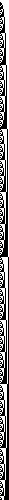Binary Progression: The numbers from 1 to 500, converted into binary and drawn as black and white squares. Each number is a series of 1s and 0s, and the 1s are drawn as black squares and the 0s as white squares.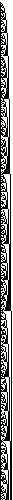Binary Primes: The prime numbers between 1 and 5000 (I think), graphed in the same way as above. I was kind of disappointed by this, honestly. I was kind of hoping all the squares would spell out “CONGRATULATIONS, YOU FOUND A PATTERN IN THE PRIMES” or something.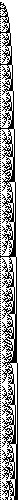Binary Squares: The squares of every number between 1 and 500. Note the interesting fractal pattern.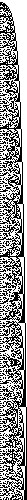Binary Cubes: The numbers from 1 to 500, cubed and displayed in binary. Notice how this one looks a lot more chaotic than did the last one.

## Visual Numbers #2Factors: The numbers from 1 to 500 are plotted horizontally across the top row. Along each vertical column, if N divides the number X (represented here by distance across the top row) evenly (that is, if N is a factor of X), then the pixel N pixels down from the top is black.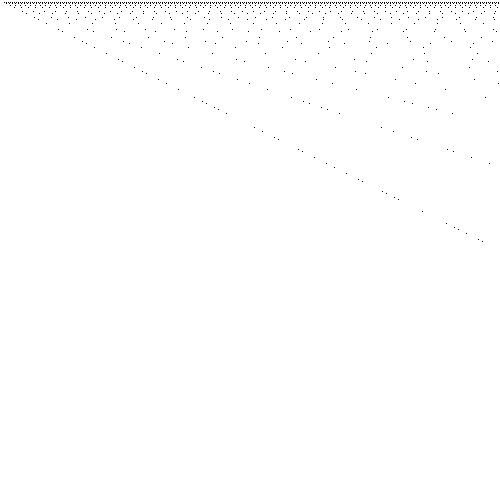Prime Factors: The same general principle as above, but in this image, only the prime factors are shown.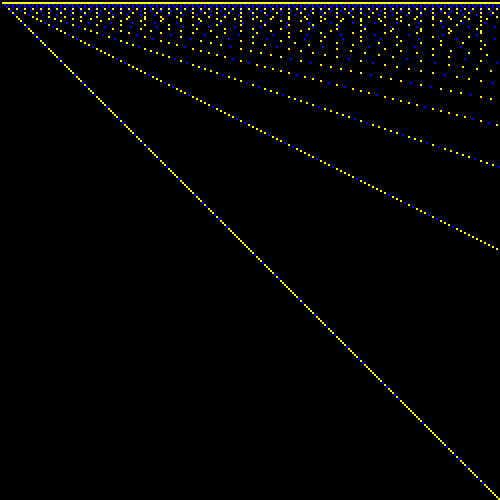Blue Over Yellow: Basically, a combination of the previous two images. Numbers from 1 to 250 are plotted horizontally, and factors are plotted vertically. If a factor is prime, the little square representing it is blue, otherwise, it’s yellow.

That tantalizing structure is still just slightly out of reach…Oh well, back to work!

## Visual Numbers #1

This is the beginning of what I hope will be a fairly long-running series of posts, each containing one or two (or three, if I’m feeling adventurous) numerical or mathematical visualizations. If you need a concrete example of what I’m talking about, just check out the image here).

Anyway, here goes: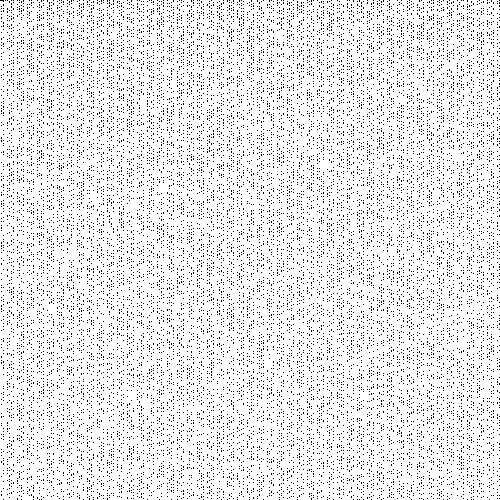Meet the Primes: Every pixel represents a number from 1 to 250,000. The image wraps horizontally; that is, the first pixel of the first row is the number 1, the first pixel of the second row is 501 (since each row is 500 pixels wide), the first pixel of the third row is 101, and so on and so on. Pixels representing prime numbers are black. From this view, it’s quite obvious that there’s likely some sort of structure to the primes, but it’s hard to say what that structure might be.